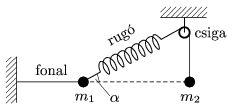Mathematical and Physical Journal
for High Schools
Issued by the MATFUND Foundation
 Already signed up? New to KöMaL?

#Problem P. 4520. (March 2013)

P. 4520. The bodies arranged as shown in the figure are at rest; m1=1 kg,=30o. The rope attached to the body of mass m1 is horizontal.

a) Determine the magnitude of m2.

b) Suppose that the rope which fixes the body of mass m1 brakes. What is the acceleration of the bodies when they begin to move?(4 pont)

Deadline expired on April 10, 2013.

Sorry, the solution is available only in Hungarian. Google translation

Megoldásvázlat. $\displaystyle a)$ $\displaystyle m_2=2m_1=2$ kg.

$\displaystyle b)$ $\displaystyle a_1=\sqrt{3}g\approx17~\rm m/s^2$; $\displaystyle a_2=0.$

### Statistics:

 130 students sent a solution. 4 points: 81 students. 3 points: 23 students. 2 points: 22 students. 1 point: 2 students. 0 point: 2 students.

Problems in Physics of KöMaL, March 2013